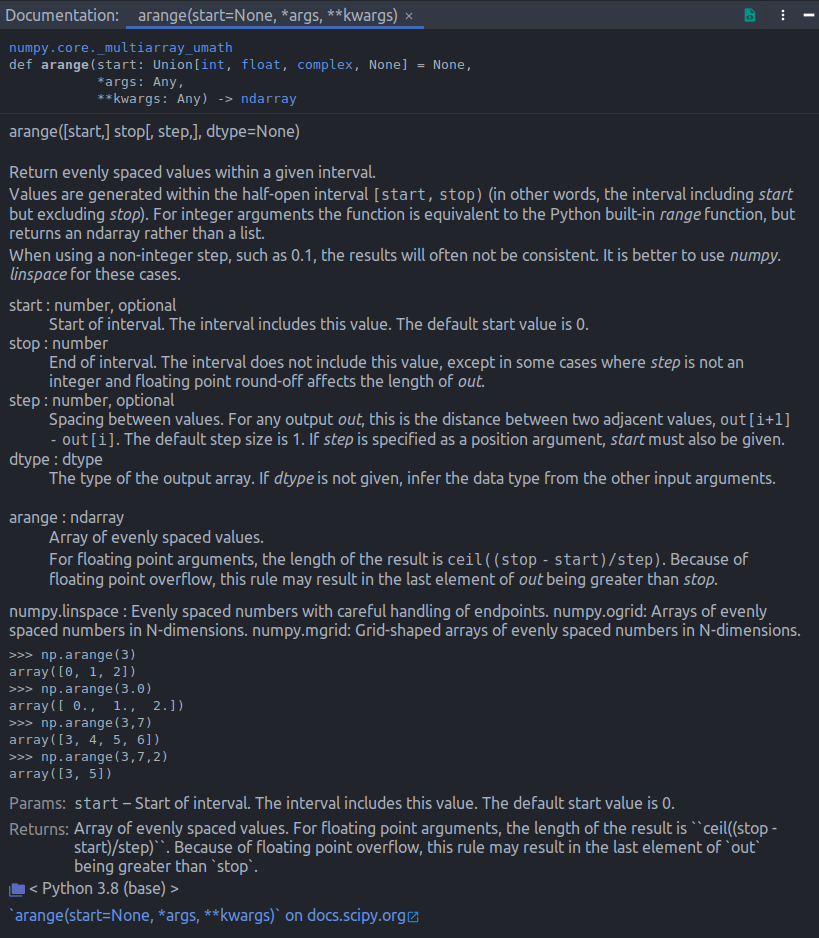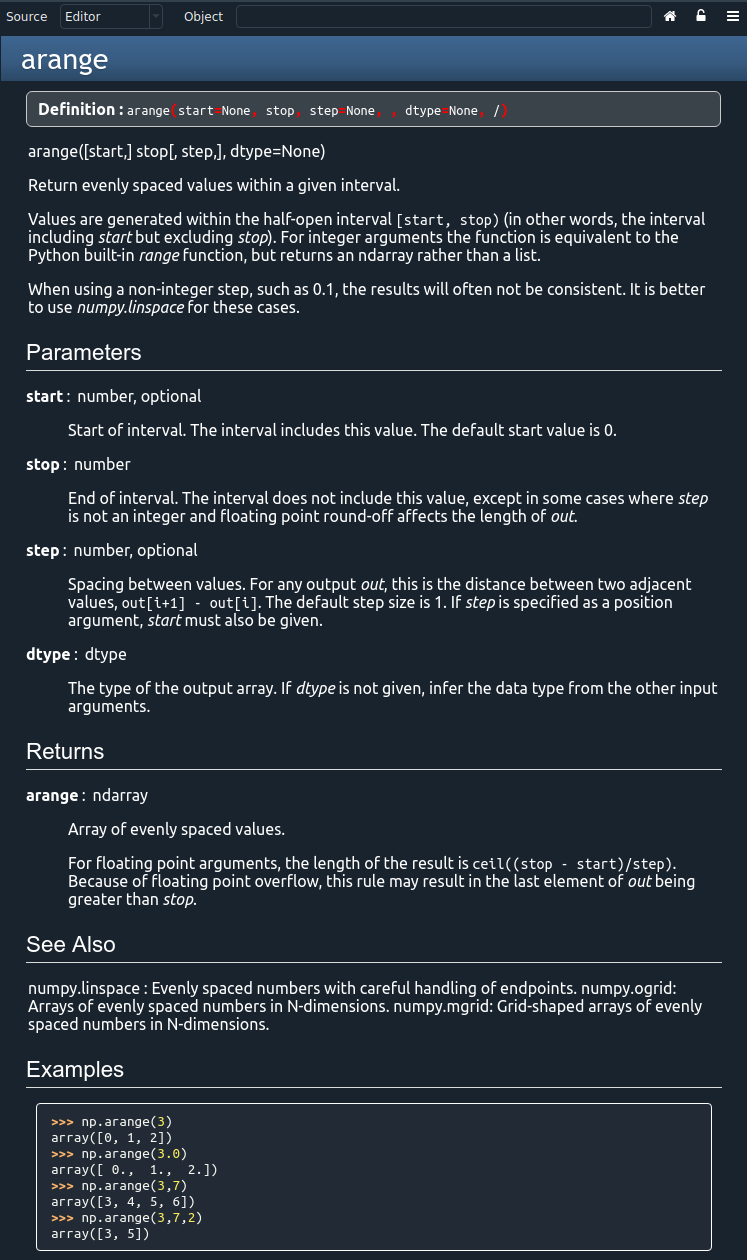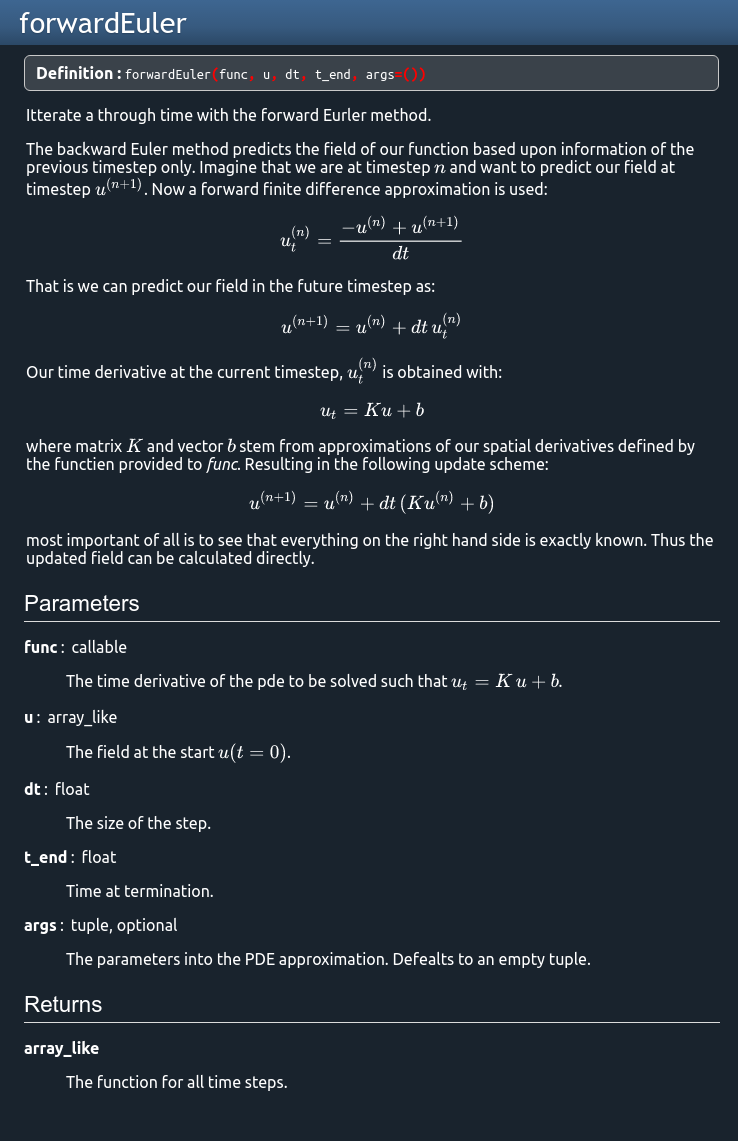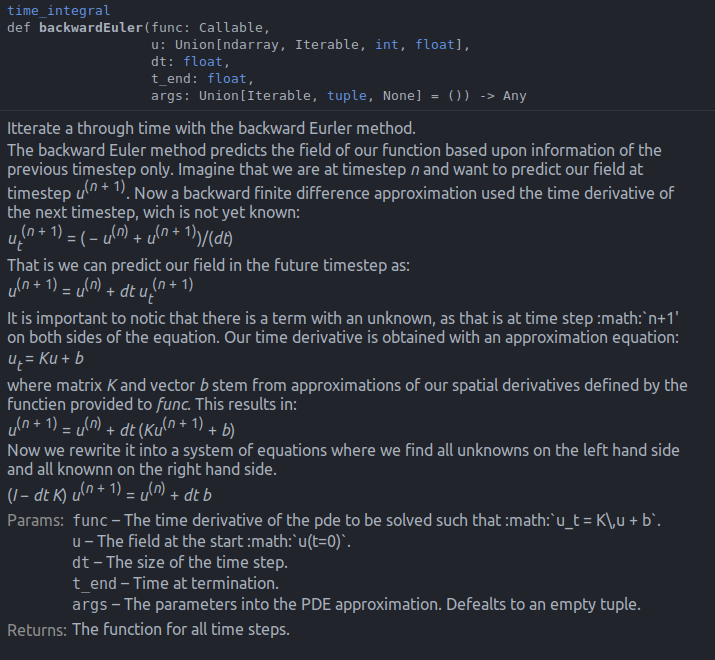Dear All,

Intro:
I'm one of those that moved from Spyder to PyCharm, I'm quite happy as it is, but there are some things that I would like to set up slightly different in PyCharm. I hope that you can help me with this.

Description:
The PyCharm documentation window is not as displayed as 'pretty' as I would like it to be. Take here an example from numpy.arange that looks like:Whereas the documentation in Spyder is more readable:I do like the increased information density in PyCharm, but it would be nice to have:

• Headers separating the different parts of the documentation.
• The use of bold for important parts such as the names of variables.
• Example code using syntax highlighting with colours.

I assume that this is possible, but I'm unaware of how to do this.

Some extra requests, Math in docsting:
There are also some more niche things that I would like to do, but I doubt that this is possible . I do use numpy style for my own docstrings and because I work on mathematics I do like to show functions in my docstrings. See here my forwardEuler doc in spyder:In PyCharm this is just not displayed as nicely. The math is rendered through restructured text (sphinx) latex support and here below is the actual code:

def backwardEuler(func, u, dt, t_end, args=()):    r"""        Itterate a through time with the backward Eurler method.        The backward Euler method predicts the field of our function based upon        information of the previous timestep only. Imagine that we are at timestep        :math:n and want to predict our field at timestep :math:u^{(n+1)}.        Now a backward finite difference approximation used the time derivative        of the next timestep, wich is not yet known:        .. math::            u^{(n+1)}_t = \frac{ -u^{(n)} + u^{(n+1)} }{dt}        That is we can predict our field in the future timestep as:        .. math::            u^{(n+1)} = u^{(n)} + dt\, u^{(n+1)}_t        It is important to notic that there is a term with an unknown, as that is        at time step :math:n+1' on both sides of the equation.        Our time derivative is obtained with an approximation equation:        .. math::            u_t = K u + b        where matrix :math:K and vector :math:b stem from approximations of our        spatial derivatives defined by the functien provided to func. This        results in:        .. math::            u^{(n+1)} = u^{(n)} + dt\, ( K u^{(n+1)} + b )        Now we rewrite it into a system of equations where we find all unknowns        on the left hand side and all knownn on the right hand side.        .. math::            (I - dt\,K)\, u^{(n+1)} = u^{(n)} + dt\,b        Parameters        ----------        func : callable            The time derivative of the pde to be solved such that :math:u_t = K\,u + b.        u : array_like            The field at the start :math:u(t=0).        dt : float            The size of the time step.        t_end : float            Time at termination.        args : tuple, optional            The parameters into the PDE approximation. Defealts to an empty tuple.        Returns        -------        array_like            The function for all time steps.        """    # The t derivative matrix is constant, as it is expensive to build these    # kind of matrices we make it only once.    K, b = func(*args)    A = sparse.identity(len(b)) - dt*K    # Update the timesteps with the implicit scheme.    max_iter = int(t_end / dt)    for n in range(max_iter):        u = spsolve(A, u + dt*b)    return u`

Summary:

1. Is there a way to make the printing of the documentation more readable. (Headers, sections, spacing and syntax)
2. Is there any way to produce better math support (which I assume should go through shpinx on docutils)?Hi, I created 3 tickets in PyCharm's bug tracker based on your feedback:

• PY-47742 Better ".. math::" blocks rendering
• PY-47743 Better block separation in quick documentation
• PY-47744 Better doctest rendering in Examples

Please feel free to create more if I missed something.

Unfortunately, I don't think it is possible to tweak the quick-documentation visual style via settings.